# De Morgan's First Law

De Morgan’s Law states that the complement of the union of two sets is the intersection of their complements and the complement of the intersection of two sets is the union of their complements. These are mentioned after the great mathematician De Morgan. This law can be expressed as ( A ∪ B) ‘ = A ‘ ∩ B ‘. In set theory, these laws relate the intersection and union of sets by complements.

## De Morgan’s Laws Statement and Proof

A well-defined collection of objects or elements is known as a set. Various operations like complement of a set, union and intersection can be performed on two sets. These operations and their usage can be further simplified using a set of laws known as De Morgan’s Laws. These are very easy and simple laws.

Any set consisting of all the objects or elements related to a particular context is defined as a universal set. Consider a universal set U such that A and B are the subsets of this universal set.

According to De Morgan’s first law, the complement of the union of two sets A and B is equal to the intersection of the complement of the sets A and B.

(A∪B)’= A’∩ B’      —–(1)

Where complement of a set is defined as

A’= {x:x ∈ U and x ∉ A}

Where A’ denotes the complement.

This law can be easily visualized using Venn Diagrams.

The L.H.S of the equation 1 represents the complement of union of two sets A and B. First of all, union of two sets A and B is defined as the set of all elements which lie either in set A or in set B. It can be visualized using Venn Diagrams as shown: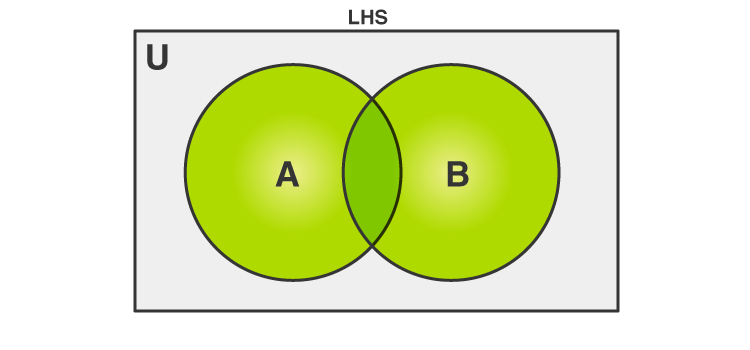Figure 1 Union of Sets

The highlighted or the green colored portion denotes A∪B. The complement of union of A and B i.e., (A∪B)’is set of all those elements which are not in A∪B. This can be visualized as follows: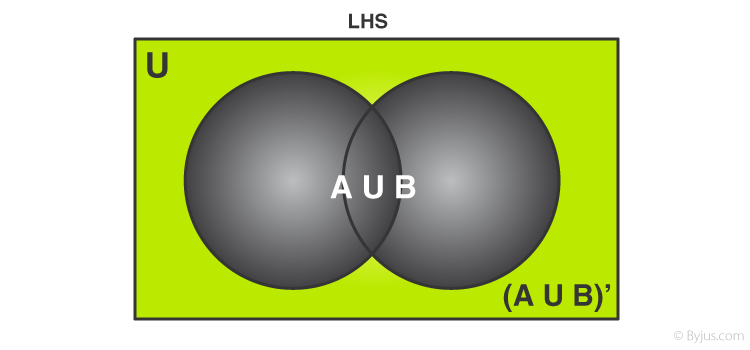Figure 2 Complement of sets

Similarly, R.H.S of equation 1 can be represented using Venn Diagrams as well, the first part i.e., A’ can be depicted as follows: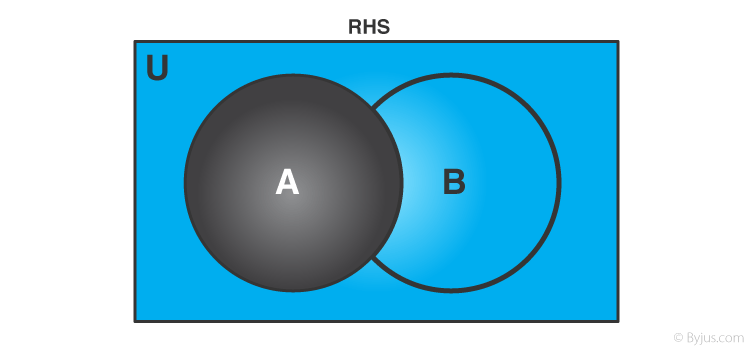Figure 3 Complement of set A

The portion in black indicates set A and the blue part denotes its complement i.e., A’.
Similarly, B’ is represented as: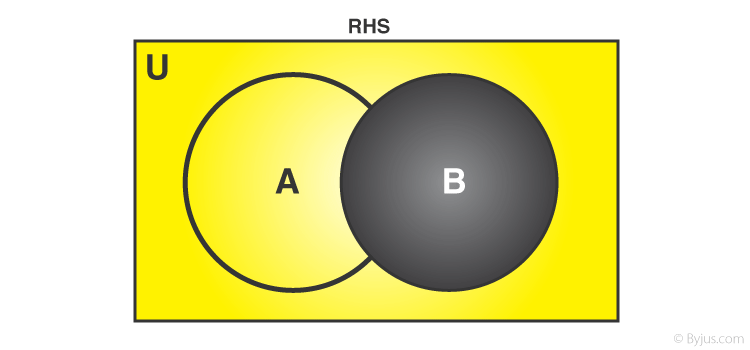Figure 4 Complement of set B

The portion in black indicates set B and the yellow part denotes its complement i.e., B’.

If fig. 3 and 4 are superimposed on one another, we get the figure similar to that of the complement of sets.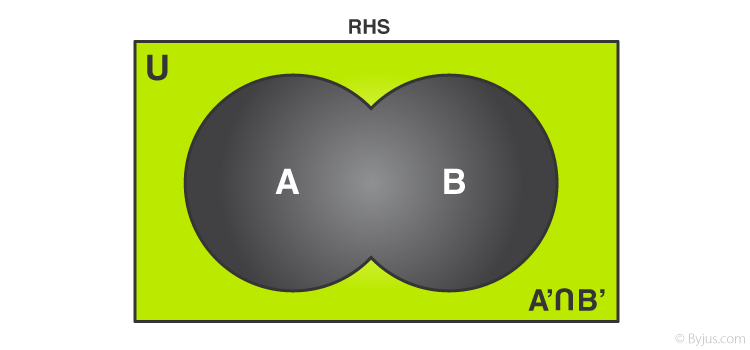Figure 5 Intersection of complements of sets

Hence L.H.S = R.H.S

Mathematically,

As, A∪B= either in A or in B

(A∪B)’= L.H.S = neither in A nor in B

Also, A’= Not in A

B’= Not in B

A’∩ B’= Not in A and not in B

⇒(A∪B)’= A’∩ B’

Thus, by visualizing the Venn Diagrams and analyzing De Morgan’s Laws by writing it down, its validity can be justified.

We may apply De Morgan’s theorem to negating a dis-junction or the negation of conjunction in all or part of a formula. This theorem explains that the complement of all the terms’ product is equal to the sum of each term’s complement. Similarly, the complement of the sum of all the terms is equal to the product of the complement of each term. Also,this theorem is used to solve different problems in boolean algebra.

1. Rajkumar

Good graphic method

2. Hikmat

Hello Sir
Please answer these questions for me i really need it right now.
Question 1: Prove the DeMorgan law A={1,2,3,4), B=(3,4,5,6}?
Question 2: Find the Power set of A={0,1,2,3,4,5,6}